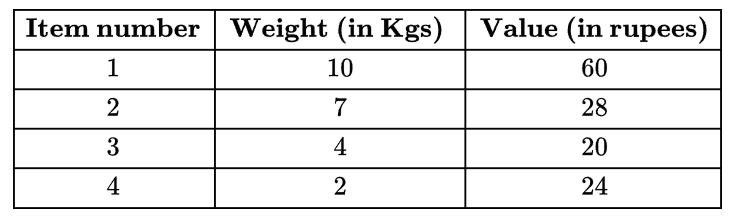# GATE | GATE CS 2018 | Question 60

Consider the weights and values of items listed below. Note that there is only one unit of each item.Question

The task is to pick a subset of these items such that their total weight is no more than 11 Kgs and their total value is maximized. Moreover, no item may be split. The total value of items picked by an optimal algorithm is denoted by Vopt. A greedy algorithm sorts the items by their value-to-weight ratios in descending order and packs them greedily, starting from the first item in the ordered list. The total value of items picked by the greedy algorithm is denoted by Vgreedy. The value of Vopt − Vgreedy is ______ .

(A)

16

(B)

8

(C)

44

(D)

60